Length

A numerical characteristic of the extension of lines in a metric space. The length of a segment of a straight line is the distance between its ends, measured by means of some segment accepted as the unit length. The length of a broken line is the sum of the lengths of its parts. The length of a simple arc is the least upper bound of the lengths of the broken lines inscribed in this arc. Any continuous curve has a length, finite or infinite. If its length is finite, the curve is known as rectifiable. The length of a planar curve defined in rectangular coordinates by an equation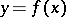,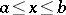(having a continuous derivative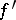) is given by the integral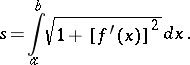If the curve is given in parametric formits length is given by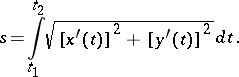The length of a rectifiable curve does not depend on the parametrization. The length of a spatial curve given in parametric form,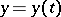,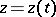,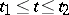, is given by the formula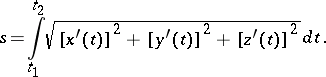In the case of an-dimensional space,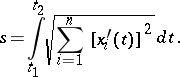Letbe a continuously-differentiable curve, given by functions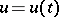,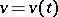, on a continuously-differentiable surface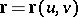. Then the length of an arc of the curve counted from the point corresponding to the parameter valueis equal to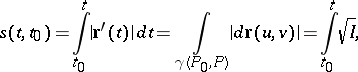whereis the first fundamental form of the surface. The length of a continuously-differentiable curve given by functions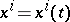,, in a Riemannian space with metric tensor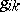is